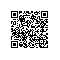# 还记得 Flappy Bird 么？这篇文章教你如何用神经网络破朋友圈纪录！

Flappy Bird（非官方译名：笨鸟先飞）是一款2013年鸟飞类游戏，由越南河内独立游戏开发者阮哈东（Dong Nguyen）开发，另一个独立游戏开发商GEARS Studios发布。—— 以上内来自《维基百科》

Flappy Bird操作简单，通过点击手机屏幕使Bird上升，穿过柱状障碍物之后得分，碰到则游戏结束。由于障碍物高低不等，控制Bird上升和下降需要反应快并且灵活，要得到较高的分数并不容易，笔者目前最多得过10分。

1. Flappy Bird 游戏展示

2. 模型：卷积神经网络

3. 算法：Deep Q Network

4. 代码：TensorFlow实现

## 一、Flappy Bird 游戏展示● 加载CUDA运算库● TensorFlow运行设备 /gpu:0TensorFlow运行设备/gpu:0

/gpu:0 这是TensorFlow平台默认的配置方法，表示使用系统中的第一块显卡。

## 二、模型：卷积神经网络sigmoid函数tanh双曲正切函数

1. 局部感知域（稀疏连接）；

2. 参数共享；

3. 多卷积核；

4. 池化。

● 1. 局部感知域（稀疏连接）

1. 需要训练的参数过多，容器导致结果不收敛（梯度消失），且训练难度极大；

2. 实际上对于某个局部的神经元来讲，它更加敏感的是小范围内的输入，换句话说，对于较远的输入，其相关性很低，权值也就非常小。● 2. 参数共享● 3. 多卷积核● 4. 池化1. 初始输入四幅图像80×80×4（4代表输入通道，初始时四幅图像是完全一致的），经过卷积核8×8×4×32（输入通道4，输出通道32），步距为4（每步卷积走4个像素点），得到32幅特征图（feature map），大小为20×20；

2. 将20×20的图像进行池化，池化核为2×2，得到图像大小为10×10；

3. 再次卷积，卷积核为4×4×32×64，步距为2，得到图像5×5×64；

4. 再次卷积，卷积核为3×3×64*64，步距为2，得到图像5×5×64，虽然与上一步得到的图像规模一致，但再次卷积之后的图像信息更为抽象，也更接近全局信息；

5. Reshape，即将多维特征图转换为特征向量，得到1600维的特征向量；

6. 经过全连接1600×512，得到512维特征向量；

7. 再次全连接512×2，得到最终的2维向量[0,1]和[1,0]，分别代表游戏屏幕上的是否点击事件。

## 三、算法：Deep Q Network

Playing Atari with Deep Reinforcement Learning

DeepMind团队通过强化学习，完成了20多种游戏，实现了端到端的学习。其用到的算法就是Q Network。2015年，DeepMind团队在《Nature》上发表了一篇升级版：

Human-level control through deep reinforcement learning● 智能代理（学习系统）

● 环境

● 马尔科夫决策过程MDP：马尔科夫决策过程

HMM（马尔科夫模型）在语音识别，行为识别等机器学习领域有较为广泛的应用。条件随机场模型（Conditional Random Field）则用于自然语言处理。两大模型是语音识别、自然语言处理领域的基石。● 贝尔曼方程● 策略迭代● 值迭代● Q-Learning

Q-Learning是根据值迭代的思路来进行学习的。该算法中，Q值更新的方法如下：Q值更新方法Q-Learning算法Q-Learning算法的过程就是存储Q值的过程。上表中，横列为状态s，纵列为Action a，s和a决定了表中的Q值。

● 第一步：初始化，将表中的Q值全部置0；

● 第二步：根据策略及状态s，选择a执行。假定当前状态为s1，由于初始值都为0，所以任意选取a执行，假定这里选取了a2执行，得到了reward为1，并且进入了状态s3。根据Q值更新公式：Q值更新公式Q值更新公式Q值

Q表格就变成：● 第三步：继续循环操作，进入下一次动作，当前状态是s3，假设选择动作a3，然后得到reward为2，状态变成s1，那么我们同样进行更新：Q值更新● 第四步： 继续循环，Q值在试验的同时反复更新，直到收敛。● Q-networkQ-networkDQN损失函数（来源于论文）DQN算法（来源于论文）

## 四、代码：TensorFlow实现

● GameState游戏类，frame_step方法控制移动

● CNN模型构建

● OpenCV-Python图像预处理方法

● 模型训练过程

1. GameState游戏类及frame_step方法

if input_actions == 1:
if self.playery > -2 * PLAYER_HEIGHT:
self.playerVelY = self.playerFlapAcc
self.playerFlapped = True
# SOUNDS['wing'].play()

frame_step方法的返回值是：

return image_data, reward, terminal

2. CNN模型构建

# 权重
def weight_variable(shape):
initial = tf.truncated_normal(shape, stddev=0.01)
return tf.Variable(initial)
# 偏置
def bias_variable(shape):
initial = tf.constant(0.01, shape=shape)
return tf.Variable(initial)
# 卷积
def conv2d(x, W, stride):
return tf.nn.conv2d(x, W, strides=[1, stride, stride, 1], padding="SAME")
# 池化
def max_pool_2x2(x):
return tf.nn.max_pool(x, ksize=[1, 2, 2, 1], strides=[1, 2, 2, 1], padding="SAME")

def createNetwork():
# 第一层卷积
W_conv1 = weight_variable([8, 8, 4, 32])
b_conv1 = bias_variable()
# 第二层卷积
W_conv2 = weight_variable([4, 4, 32, 64])
b_conv2 = bias_variable()
# 第三层卷积
W_conv3 = weight_variable([3, 3, 64, 64])
b_conv3 = bias_variable()
# 第一层全连接
W_fc1 = weight_variable([1600, 512])
b_fc1 = bias_variable()
# 第二层全连接
W_fc2 = weight_variable([512, ACTIONS])
b_fc2 = bias_variable([ACTIONS])

# 输入层
s = tf.placeholder("float", [None, 80, 80, 4])

# 第一层隐藏层+池化层
h_conv1 = tf.nn.relu(conv2d(s, W_conv1, 4) + b_conv1)
h_pool1 = max_pool_2x2(h_conv1)
# 第二层隐藏层（这里只用了一层池化层）
h_conv2 = tf.nn.relu(conv2d(h_pool1, W_conv2, 2) + b_conv2)
# h_pool2 = max_pool_2x2(h_conv2)
# 第三层隐藏层
h_conv3 = tf.nn.relu(conv2d(h_conv2, W_conv3, 1) + b_conv3)
# h_pool3 = max_pool_2x2(h_conv3)
# Reshape
# h_pool3_flat = tf.reshape(h_pool3, [-1, 256])
h_conv3_flat = tf.reshape(h_conv3, [-1, 1600])
# 全连接层
h_fc1 = tf.nn.relu(tf.matmul(h_conv3_flat, W_fc1) + b_fc1)
# 输出层
readout = tf.matmul(h_fc1, W_fc2) + b_fc2

return s, readout, h_fc1

3. OpenCV-Python图像预处理方法

x_t, r_0, terminal = game_state.frame_step(do_nothing)
# 首先将图像转换为80*80，然后进行灰度化
x_t = cv2.cvtColor(cv2.resize(x_t, (80, 80)), cv2.COLOR_BGR2GRAY)
# 对灰度图像二值化
ret, x_t = cv2.threshold(x_t, 1, 255, cv2.THRESH_BINARY)
# 四通道输入图像
s_t = np.stack((x_t, x_t, x_t, x_t), axis=2)

4. DQN训练过程

i. 在进入训练之前，首先创建一些变量：

# define the cost function
a = tf.placeholder("float", [None, ACTIONS])
y = tf.placeholder("float", [None])

# open up a game state to communicate with emulator
game_state = game.GameState()

# store the previous observations in replay memory
D = deque()

# perform gradient step
train_step.run(feed_dict={
y: y_batch,
a: a_batch,
s: s_j_batch}
)

ii. 创建游戏及经验池 D

# open up a game state to communicate with emulator
game_state = game.GameState()

# store the previous observations in replay memory
D = deque()

# Create two variables.
weights = tf.Variable(tf.random_normal([784, 200], stddev=0.35),
name="weights")
biases = tf.Variable(tf.zeros(), name="biases")
...
# Add an op to initialize the variables.
init_op = tf.global_variables_initializer()

# Later, when launching the model
with tf.Session() as sess:
# Run the init operation.
sess.run(init_op)
...
# Use the model
...

iii. 参数保存及加载

saver = tf.train.Saver()

# Create some variables.
v1 = tf.Variable(..., name="v1")
v2 = tf.Variable(..., name="v2")
...
# Add ops to save and restore all the variables.
saver = tf.train.Saver()

# Later, launch the model, use the saver to restore variables from disk, and
# do some work with the model.
with tf.Session() as sess:
# Restore variables from disk.
saver.restore(sess, "/tmp/model.ckpt")
print("Model restored.")
# Do some work with the model
...

# saving and loading networks
saver = tf.train.Saver()
checkpoint = tf.train.get_checkpoint_state("saved_networks")
if checkpoint and checkpoint.model_checkpoint_path:
saver.restore(sess, checkpoint.model_checkpoint_path)
else:
print("Could not find old network weights")

# save progress every 10000 iterations
if t % 10000 == 0:
saver.save(sess, 'saved_networks/' + GAME + '-dqn', global_step=t)

iv. 实验及样本存储

# choose an action epsilon greedily
a_t = np.zeros([ACTIONS])
action_index = 0
if t % FRAME_PER_ACTION == 0:
if random.random() <= epsilon:
print("----------Random Action----------")
action_index = random.randrange(ACTIONS)
a_t[random.randrange(ACTIONS)] = 1
else:
a_t[action_index] = 1
else:
a_t = 1  # do nothing

# run the selected action and observe next state and reward
x_t1_colored, r_t, terminal = game_state.frame_step(a_t)
x_t1 = cv2.cvtColor(cv2.resize(x_t1_colored, (80, 80)), cv2.COLOR_BGR2GRAY)
ret, x_t1 = cv2.threshold(x_t1, 1, 255, cv2.THRESH_BINARY)
x_t1 = np.reshape(x_t1, (80, 80, 1))
# s_t1 = np.append(x_t1, s_t[:,:,1:], axis = 2)
s_t1 = np.append(x_t1, s_t[:, :, :3], axis=2)

# store the transition in D
D.append((s_t, a_t, r_t, s_t1, terminal))

# update the old values
s_t = s_t1
t += 1

v. 通过梯度下降进行模型训练

if t > OBSERVE:
# sample a minibatch to train on
minibatch = random.sample(D, BATCH)

# get the batch variables
s_j_batch = [d for d in minibatch]
a_batch = [d for d in minibatch]
r_batch = [d for d in minibatch]
s_j1_batch = [d for d in minibatch]

y_batch = []
for i in range(0, len(minibatch)):
terminal = minibatch[i]
# if terminal, only equals reward
if terminal:
y_batch.append(r_batch[i])
else:

train_step.run(feed_dict={
y: y_batch,
a: a_batch,
s: s_j_batch}
)

s_j_batch、a_batch、r_batch、s_j1_batch是从经验池D中提取到的马尔科夫序列（Java童鞋羡慕Python的列表推导式啊），y_batch为标签值，若游戏结束，则不存在下一步中状态对应的Q值（回忆Q值更新过程），直接添加r_batch，若未结束，则用折合因子（0.99）和下一步中状态的最大Q值的乘积，添加至y_batch。

====================================分割线================================